### Damped Free Vibrations of Single Degree of Freedom Systems: Damping

In the systems we have considered so far, once a vibration has started it will continue indefinitely with energy continually being converted between its mechanical forms – kinetic and potential. Of course, real physical systems do not behave this way. In real systems, energy not only gets converted between kinetic and potential forms, but is gradually converted into other forms as well, such as heat and sound. The methods by which this process occurs are complex, but are generally referred to as damping. In a damped system, as the energy is converted from mechanical energy to other forms, the amplitude of the motion gradually decays.

The physical mechanisms behind this process are complex and we generally prefer not to have to model the details of these processes. As a result, a number of simpler models have been developed for use in common vibration systems. A few of these are discussed below.

1) Coulomb Damping (Dry Friction Damping)
This damping is due to friction between two surfaces which are dry or have little lubrication. The friction force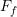is assumed to be proportional to the normal force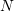between the surfaces and a coefficient of friction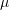,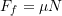The friction force is always opposed to the direction of the relative velocity between the two surfaces but is assumed to be independent of the magnitude of the relative velocity. The differential equation which describes such a system is usually linear for one–half cycles only (i.e. until the friction force changes direction).

2) Fluid Damping (Fast–Fluid Damping or Velocity–Squared Damping)
This type of damping is associated with systems which move in a fluid medium. The damping force always acts against the relative velocity and is assumed to be proportional to the relative velocity squared. Typically this is called a drag force and is given by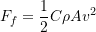Where= relative velocity,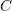= drag coefficient,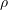= fluid density,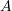= projected area of mass normal to relative velocity

3) Solid Damping (Hysteretic Damping)
This type of damping occurs within solid structures and is due to losses associated with internal friction. The friction force is typically assumed to be proportional to both displacement and velocity. This is also known as hysteretic damping because the stress–strain diagram for these materials demonstrates hysteresis when the body undergoes vibrations.

4) Viscous Damping (Slow–Fluid Damping)
Viscous damping is the most commonly used damping mechanism in vibration analysis of single degree of freedom systems. In viscous damping the force always acts to oppose the relative motion and is assumed to be proportional to the relative velocity. This is a reasonable representation of the damping mechanism in some situations:

• fluid film between two sliding surfaces (well lubricated),
• fluid film in a journal bearing,
• fluid flow through an orifice or other similar restriction,
• fluid flow past a piston in a cylinder.

In these cases, the fluid’s viscosity is an important factor. A viscous damper can be either linear or nonlinear (similar to a spring). If the damper is linear, the force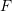due to the damping element is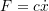whereis the damping constant and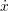is the relative velocity. Note that the units ofare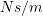. Also, note that an equivalent viscous damper can be defined using a similar procedure to that for springs.

While a viscous damper is a reasonable model of the damping in certain situations, it is often used in situations where it is not a particularly good model. There are two main reasons for this. The first is that most engineering systems possess little damping (there are exceptions of course). When there is only a small amount of damping present, most of the damping models produce qualitatively similar results. The second reason is simply that when a viscous damping model is used, the governing differential equations remain linear and therefore easier to deal with.